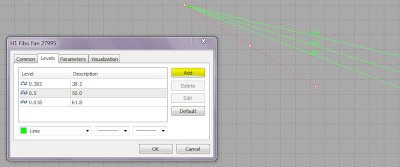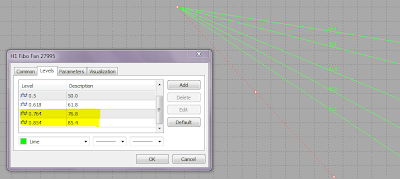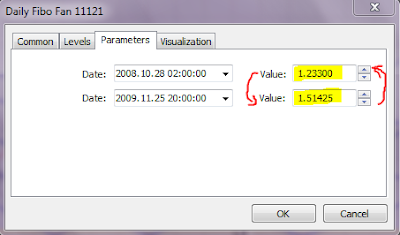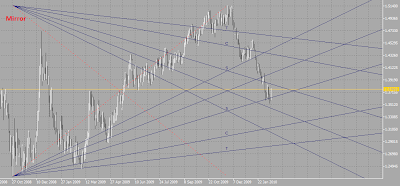## Metatrader 5 Fibonacci Tools part 2: Fibonacci Fan

This is the second article in a 5-part series about the Metatrader 5 Fibonacci Tools. In this article we are going to customize the Fibonacci Fan settings within Metatrader 5 so it can better identify major support and resistance levels. The customized Fibonacci Fan can be used also as a powerful stand-alone trading system.

In Metatrader 5 you can find the Fibonacci Fan tool under the menu Insert -> Objects -> Fibonacci -> Fibonacci Fan. First, place the Fibonacci Fan anywhere on the chart and go to the properties window:Now, add 2 more levels: 0,764 and 0,854. After adding the new levels, the fan should be like this:The main Fibonacci Fan levels are:

1: 0,382 - Signal Level
2: 0,5 - Mid-way
3: 0,618 - magnetizes the Target Level
4: 0,764 - Correction Level
5: 0,854 - Target Level

You can customize the description of within the Metatrader 5 Fibonacci Fan levels properties like this:There are 3 basic models of the price movement within the Fibonacci Fan levels.

1. Rebound model, when the price is repelled ( bounces off ) from the current target level (0,854)
2. Bolt model, when the price, before reaching the current target level bounces off a level of the Fibonacci Fan with a higher priority ( higher timeframe )
3. Breakthrough model, when the price is breaking through the current target level, because it is attracted by a level of the Fibonacci Fan with a higher priority ( higher timeframe )The price runs from the target level of the last Fibonacci Fan to the target level of current Fibonacci Fan.

A - previous fan
B - current fan
A1 - current target level (0,854)
B1 - support level and the target level for the future fan C-C1.

On the chart above you can see the Rebound model and the Breakthrough model in action. Usually, within the Bolt and Breakthrough model, the price reaches high volatility when it is reversing its course.Target Level: After the price reversal, the target is set on the target level (0,854) of the previous Fibonacci Fan ( previous wave ). If we look at current EUR/USD position, the target for the major wave will be around 1.3000 end July/begin Augustus this year. Another important instrument are the Fibonacci Arcs, with this tool we can clarify the target level within a certain time period, it is the crossing of the Fibonacci Fan's target level (0,854) with the Fibonacci Arc's 0.764 level. I'm going to write about the Fibonacci Fan & Fibonacci Arcs combination in the next article.

There is a wonderful indicator that is build for Fibonacci Fan trading, Fiba +, currently it is available only for Metatrader 4. You can download it below with the indicator template. It draws the main Fibonacci Fan levels as described above and gives them priorities. Recommended time-frame for this indicator is H4.Another important this is the Fibonacci Fan Mirror. You can create it buy reversing the price value in the properties windows of the Fan that you want to mirror:The Fibonacci Fan Mirror can be used to identify resistance levels for the current price move within the current Fibonacci Fan. For example, if the price bounces off the 0,618 level of the fan mirror, the target level of the mirror becomes a major resistance. You can also use the Fibonacci Fan Mirror for placing stop loss or lock positions ( however as we know, in Metatrader 5 it's not possible :( ), the stop loss level should be placed above the mirror's target level 0,854. I'm going to write more about this in future articles.There is also a indicator for Metatrader 4 that draws Fibonacci Fan Mirrors automatically, it works in combination with the Fibo + indicator, download it below: